﻿ 基于小波解调-1(1/2)维谱的舰船辐射噪声调制特征提取
 舰船科学技术2019, Vol. 41Issue (8): 122-126PDF

Modulation feature extraction of ship-radiated noise based on wavelet demodulation-1(1/2) D spectrum
ZHAO Mian, SONG Yu-long, ZHENG Wei
School of Electrical and Information Engineering, Jiangsu University of Science and Technogly, Zhenjiang 212003, China
Abstract: The modulation spectrum of ship-radiated noise contains a large number of inherent characteristics of the ship, which can be used to estimate the speed of ship and target classification and identification. This paper proposes a joint analysis method based on wavelet demodulation and 1(1/2)-dimensional spectrum. Firstly, the signal is demodulated by using the band-pass filtering capability of the Morlet wavelet and the signal demodulation function provided by the orthogonality of real and imaginary parts. The scale envelope spectrum is selected and the scale components containing the modulation information are selected, and 1(1/2) spectrum analysis is performed to obtain the basic frequency and harmonic information of the ship shaft frequency. The actual data results show that this method can effectively extract the modulation characteristic information in ship noise and has a good application prospect.
Key words: ship-radiated noise     wavelet demodulation     1(1/2) D spectrum     modulation feature extraction
0 引　言

1 方法原理 1.1 Morlet小波解调

Morlet小波是高斯包络下的复指数小波，其定义式为：

 ${{h}}\left( t \right) = \frac{1}{{\sqrt {{\text{π}} {f_b}} }}{e^{\left( {j2{\text{π}} {f_c}t - {t^2}/{f_b}} \right)}} {\text{，}}$ (1)

 ${{H}}\left( {{f}} \right) = {e^{ - {{\text{π}} ^2}{f_b}{{\left( {f - {f_c}} \right)}^2}}}{\text{。}}$ (2)

 ${H_i}\left( f \right) = - j{\rm{sgn}} \left( f \right){H_r}\left( f \right){\text{。}}$ (3)

 $cwt\left( {s,t} \right) = \frac{1}{s}\int_{ - \infty }^{ + \infty } {x\left( f \right)} h\left( {\frac{{t - f}}{s}} \right){\rm d}f{\text{。}}$ (4)

 $cwt\left( {s,t} \right) = x\left( t \right) * {h_s}\left( t \right){\text{，}}$ (5)

 $\begin{gathered} cwt\left( {s,t} \right) = x\left( t \right) * h_s^r\left( t \right) + x\left( t \right) * jh_s^i\left( t \right) =\\ {\rm{Re}} \left( {cwt\left( {s,t} \right)} \right) + {\rm{Im}} \left( {cwt\left( {s,t} \right)} \right){\text{。}} \end{gathered}$ (6)

 $\mu \left( {s,t} \right) = \sqrt {{\rm{Re}} {{\left( {cwt\left( {s,t} \right)} \right)}^2} + {\rm{Im}} {{\left( {cwt\left( {s,t} \right)} \right)}^2}}{\text{。}}$ (7)

1.2 1（1/2）维谱特性分析

 $R\left( {{\tau _1},{\tau _2}} \right) = E\left\{ {d\left( t \right)d\left( {t + {\tau _1}} \right)d\left( {t + {\tau _2}} \right)} \right\}{\text{，}}$ (8)

3阶累积量 $R\left( {{\tau _1},{\tau _2}} \right)$ 的对角线切片为：

 $c\left( \tau \right) = c\left( {\tau ,\tau } \right) = E\left\{ {d\left( t \right)d\left( {t + \tau } \right)d\left( {t + \tau } \right)} \right\}{\text{，}}$ (9)

$c\left( \tau \right)$ 作一维 $\rm {\text{，}}Fourier$ 变换，即可得到小波系数 $d\left( t \right)$ 的1（1/2）维谱

 $C\left( \omega \right) = \sum\limits_{\tau = - \infty }^\infty {c\left( \tau \right)} {e^{ - j\omega \tau }}{\rm d}\tau{\text{。}}$ (10)

1）去除各数据段中的直流分量；

2）参照式（9），计算各个数据的3阶累积量，并得到其对角线切片 ${c_i}\left( \tau \right)$

3）取所有数据段的 ${c_i}\left( \tau \right)$ 的均值，可到 $\mathop c\limits^ \wedge \left( \tau \right) =$ $\dfrac{1}{K}\sum\limits_{i = 1}^k {{c^{\left( i \right)}}} \left( \tau \right)$

4）对均值 $\mathop c\limits^ \wedge \left( \tau \right)$ 作一维Fourier变换，即可得到待处理信号的1（1/2）维谱。

2 舰船辐射噪声调制特征提取 2.1 调制特征提取流程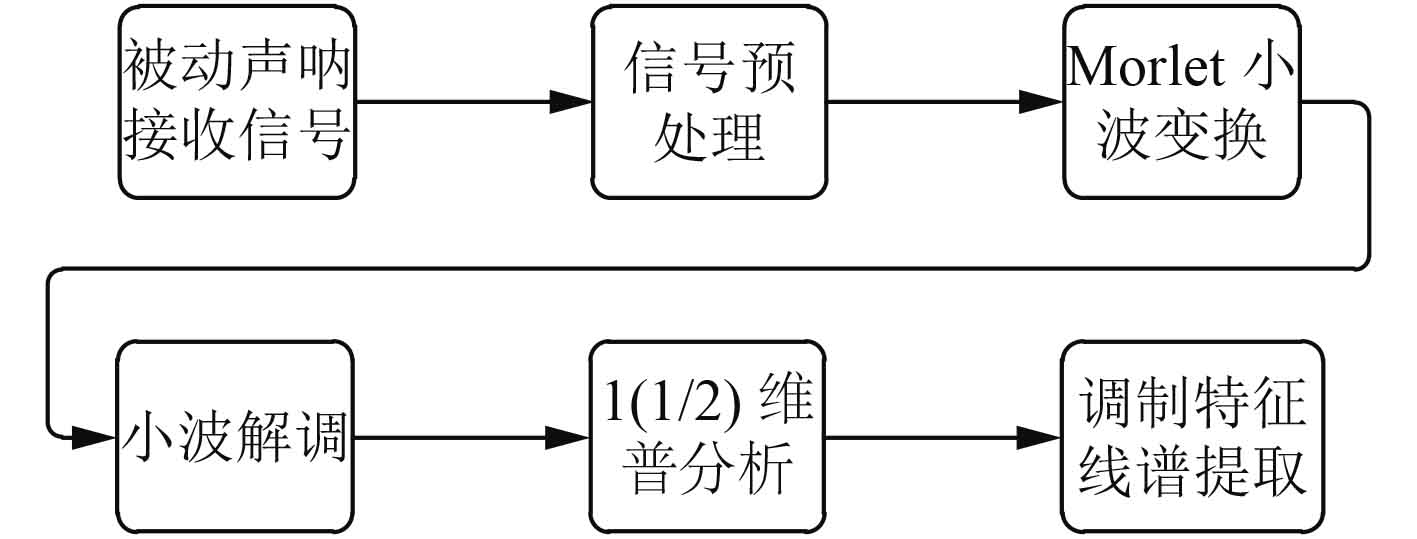图 1 工作流程图 Fig. 1 Work flow chart
2.2 特征提取方法具体实现

 ${F_a} = \frac{1}{S} * {f_c} * {f_s}{\text{，}}$ (11)

 ${S_j} = {c / {\left( {m - j} \right)}}\;\;\;j = 0,1, \cdots ,m - 1{\text{。}}$ (12)

3 实验数据处理分析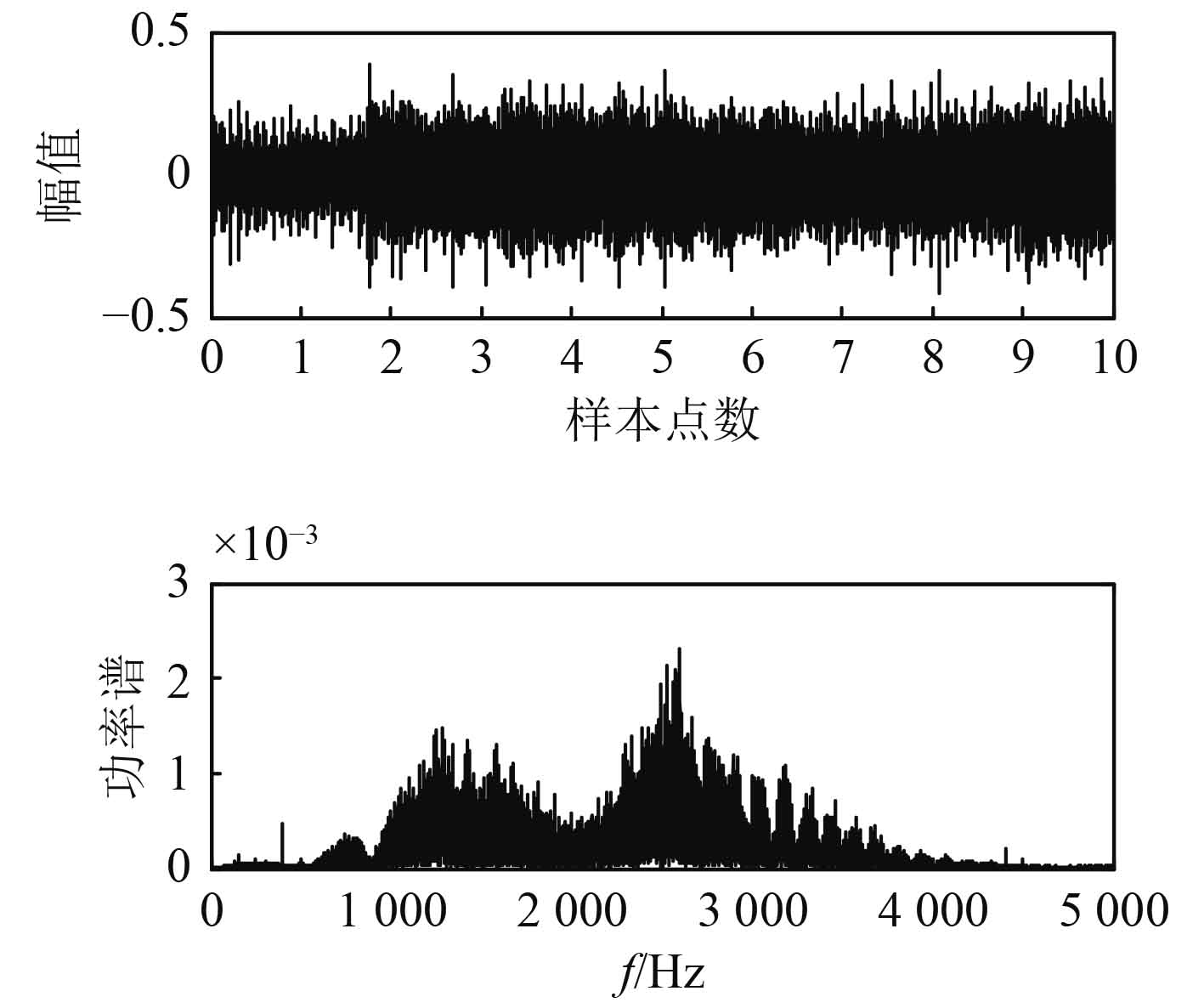图 2 舰船噪声原始信号及功率谱 Fig. 2 Ship noise original signal and power spectrum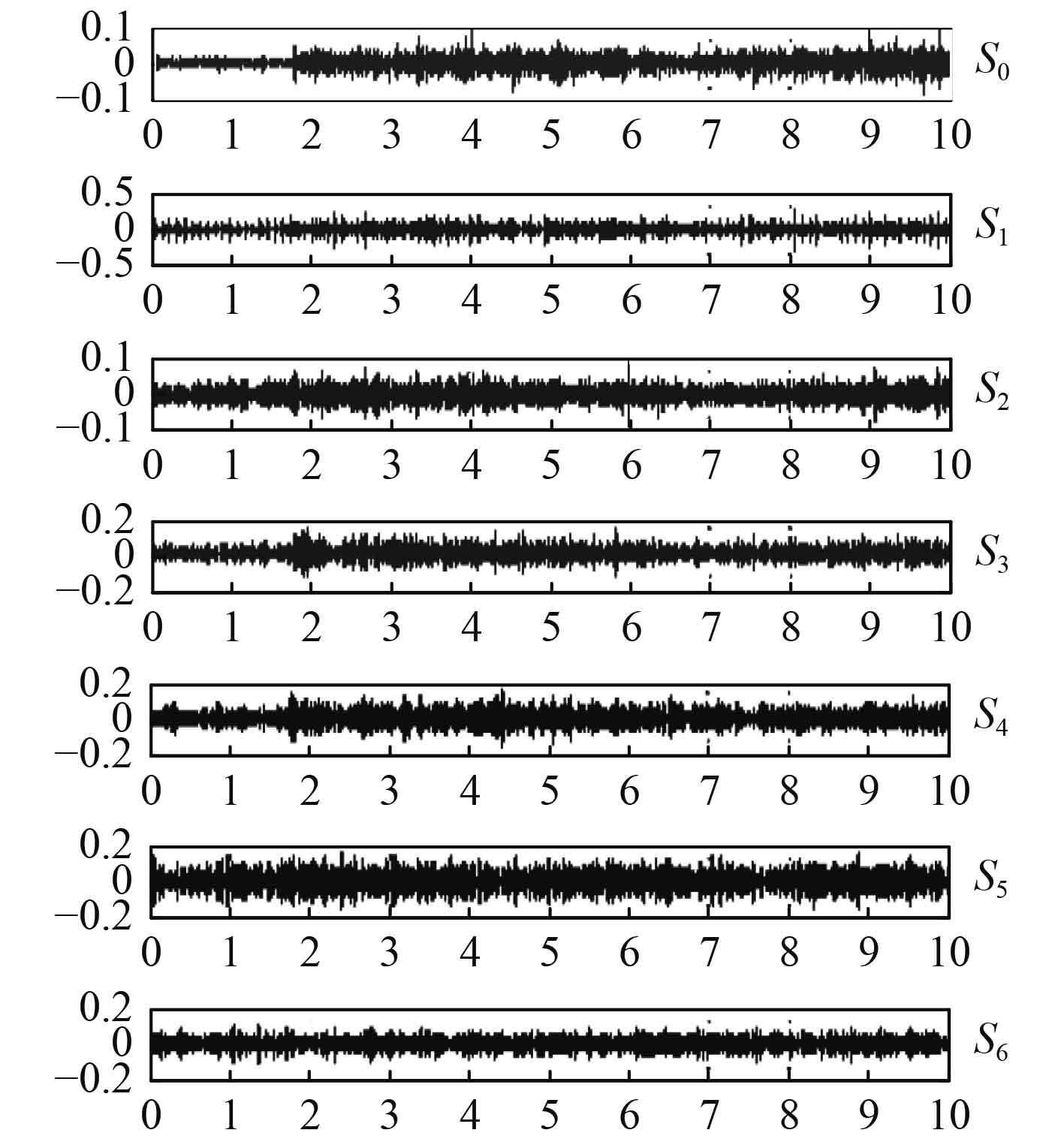图 3 Morlet小波分解图 Fig. 3 Morlet wavelet decomposition chart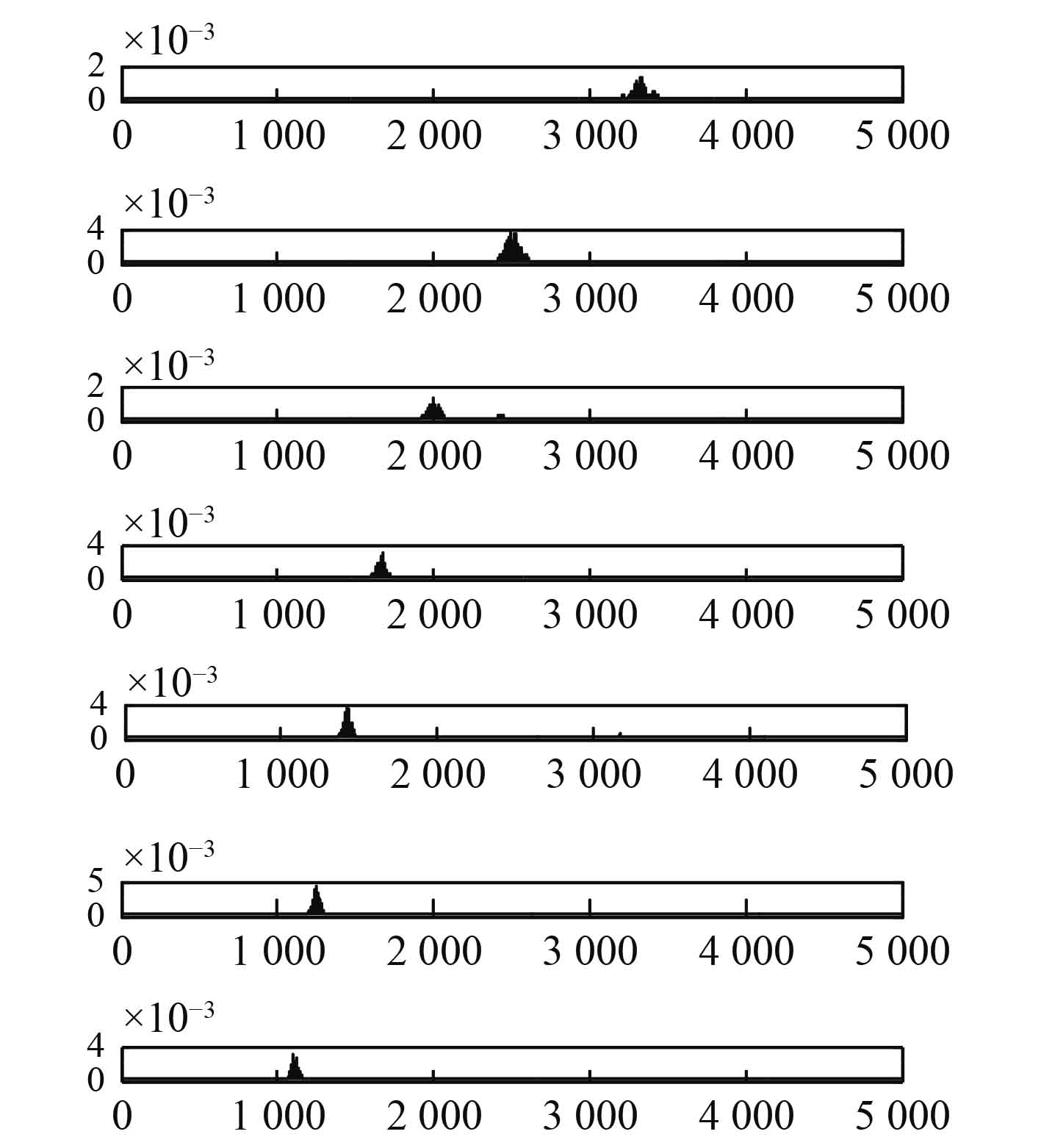图 4 小波分量功率谱 Fig. 4 Wavelet component power spectrum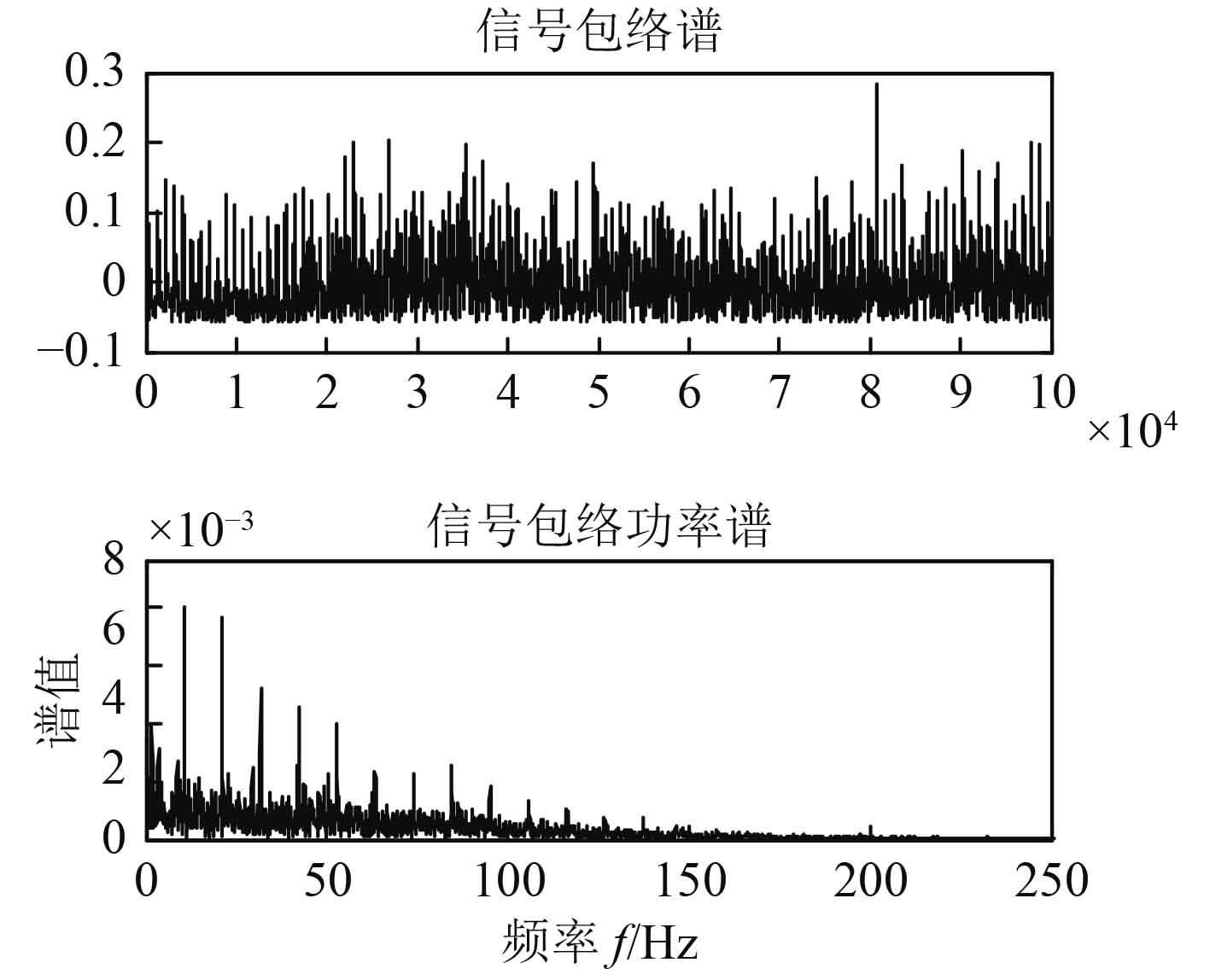图 5 信号包络及其功率谱 Fig. 5 Signal envelope and its power spectrum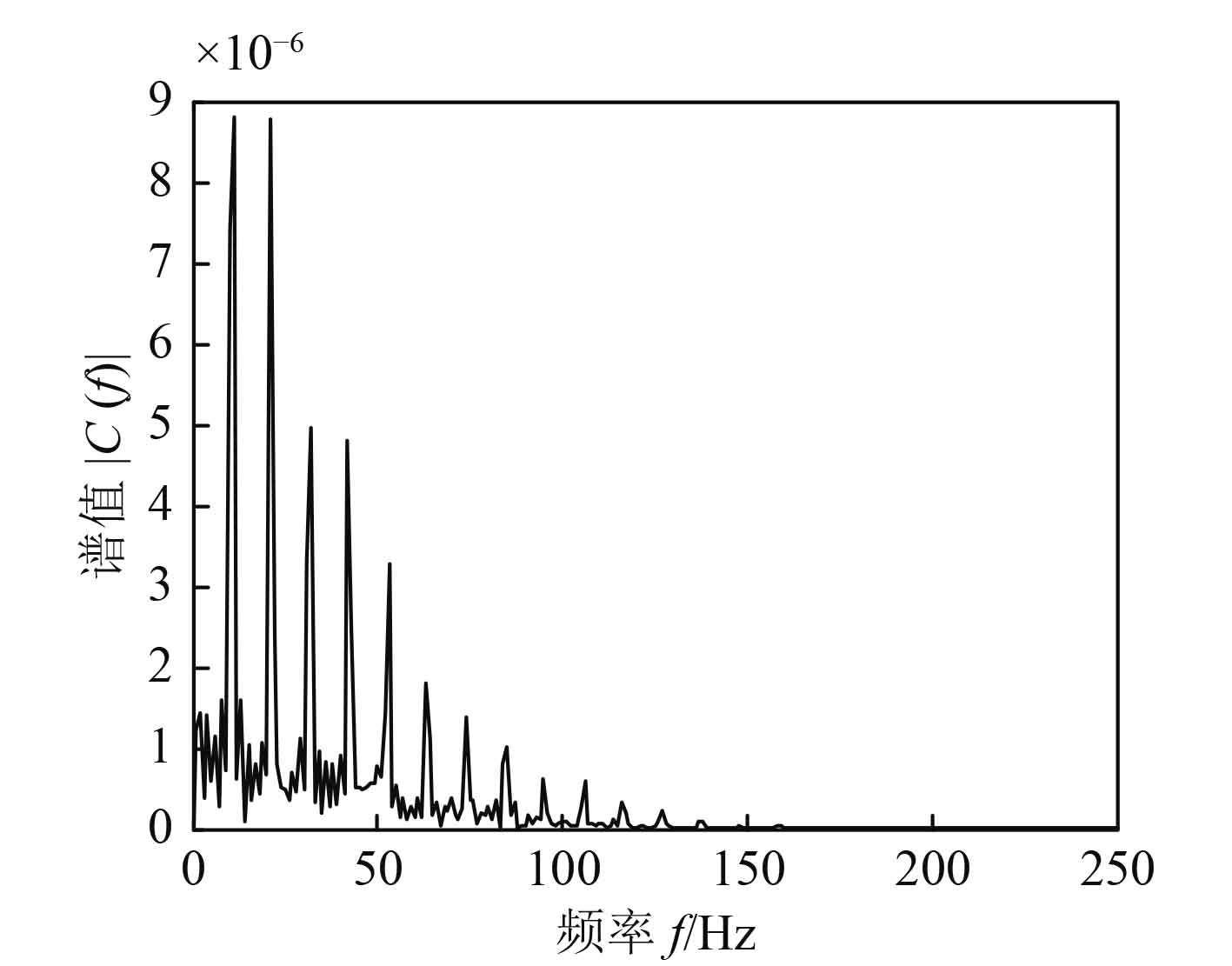图 6 1（1/2）维谱分析图 Fig. 6 1（1/2）Dspectrum analysis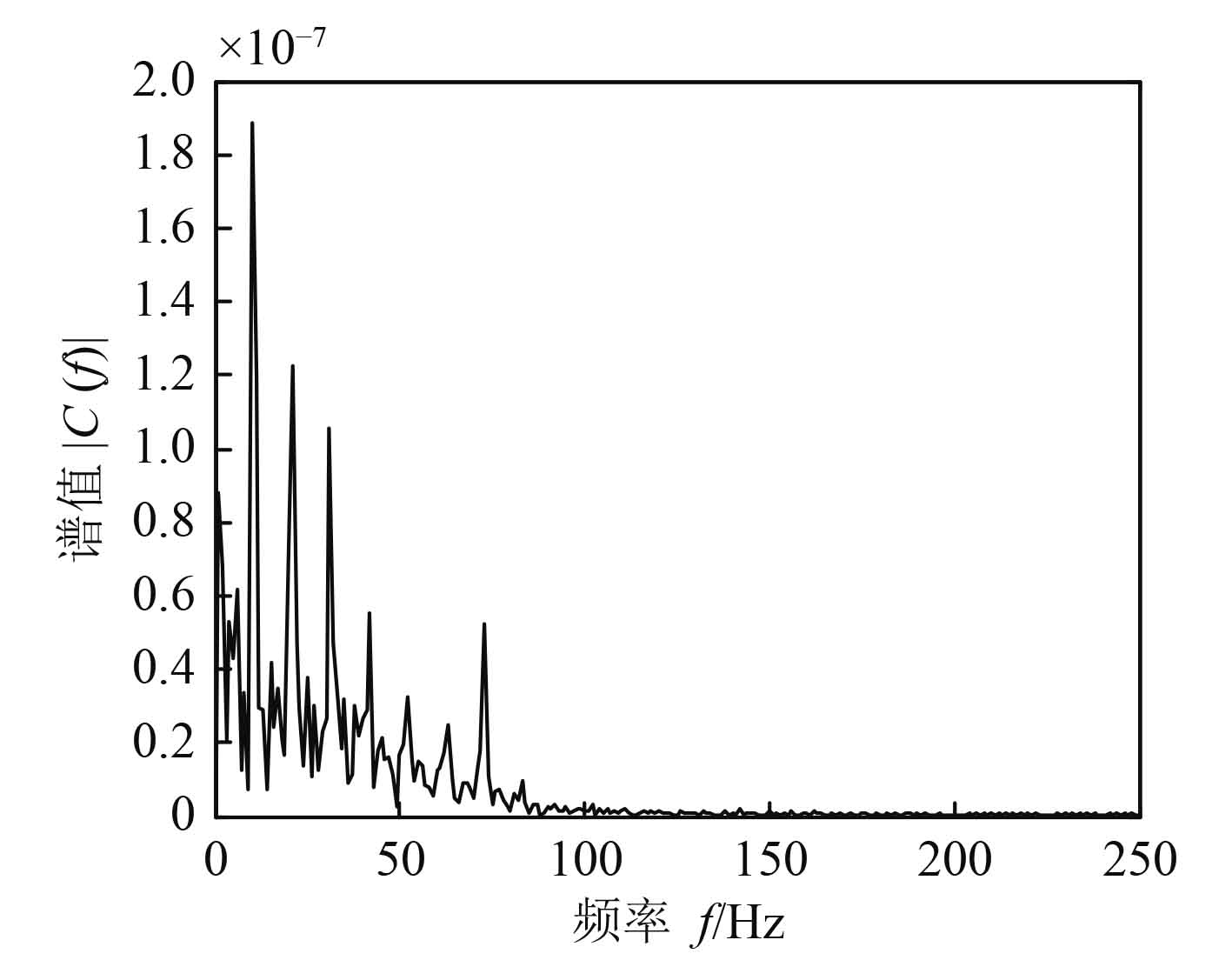图 7 不同航速下的（1/2）维谱图 Fig. 7 1（1/2）dimension spectrum at different speeds
4 结　语

  程广涛, 戴卫国, 王易川, 等. 基于小波变换的舰船辐射噪声调制特征分析[J]. 火力与指挥控制, 2007(5): 75-77+101. CHENG Guang-tao, DAI Wei-guo, WANG Yi-chuan, et al. The modulation feature analysis of ship radiated noise based on wavelet transform[J]. Fire Control and Command Control, 2007(5): 75-77+101. DOI:10.3969/j.issn.1002-0640.2007.05.021  龙燕, 温然. 舰船辐射噪声1(1/2)维谱的自适应子波特征提取[J]. 舰船科学技术, 2017, 39(14): 61-63.  LONG Yan, WEN Ran. Adaptive wavelet feature extraction of 1(1/2) dimensional spectrum of ship radiated noise[J]. Ship Science and Technology, 2017, 39(14): 61-63.  郑思仪, 郭红霞, 李亚安, 等. 一种用Duffing振子检测舰船辐射噪声线谱的新方法[J]. 科学通报, 2007(3): 258-263. ZHENG Si-yi, GUO Hong-xia, LI Ya'an, et al. A new method for detecting radiation noise line spectrum of ships using Duffing oscillator[J]. Chinese Science Bulletin, 2007(3): 258-263. DOI:10.3321/j.issn:0023-074X.2007.03.002  殷敬伟, 惠俊英, 姚直象, 等. 基于DEMON线谱的轴频提取方法研究[J]. 应用声学, 2005(6): 369-374. YIN Jing-wei, HUI Jun-ying, YAO Zhi-xiang, et al. Extraction of shaft frequency based on the DEMON line spectrum[J]. Applied Acoustics, 2005(6): 369-374. DOI:10.3969/j.issn.1000-310X.2005.06.008  何岭松, 李巍华. 用Morlet小波进行包络检波分析[J]. 振动工程学报, 2002(1): 123-126. HE Ling-song, LI Yu-hua. Envelope detection analysis with morlet wavelet[J]. Journal of Vibration Engineering, 2002(1): 123-126.  姚祺, 王彬, 张俊林. Alpha稳定分布噪声下的舰船识别分析[J]. 信号处理, 2018, 34(2): 174-182. YAO Wei, WANG Bin, ZHANG Jun-lin. The analysis of ship recognition in the alpha stable distribution noise environments[J]. Journal of Signal Processing, 2018, 34(2): 174-182.  李余兴, 李亚安, 陈晓. 基于EEMD的舰船辐射噪声特征提取方法研究[J]. 振动与冲击, 2017, 36(5): 114-119. LI Yu-xing, LI Ya'an, CHEN Xiao. Ships′ radiated noise feature extraction based on EEMD[J]. Journal of Vibration and Shock, 2017, 36(5): 114-119.  张贤达, 现代信号处理[M]. 北京: 清华大学出版社, 2015.  余桐奎, 刘文帅, 于树华, 等. 基于高阶统计量的舰船瞬态噪声测量分析方法[J]. 哈尔滨工程大学学报, 2017(9): 1-7. YU Tong-kui, LIU Wen-shuai, YU Shu-hua, et al. Measurement and analytical method for ship radiating transient noise based on high-order statistic[J]. Journal of Harbin Engineering University, 2017(9): 1-7.  AKSHADA N. K, VIDHYA M. S, RAJVEER K. S, ARNAB das. Analysis of ship noise from underwater ambient noise[J]. IEEE, Conference on Advances in Signal Processing (CASP), 2016, 265-269.  FEDERICO T, TOMASO , GIORGIO T. Parametric analysis of ship noise spectra[J]. IEEE, Journal of Oceanic Engineering, 2017, 42(2): 424-438. DOI:10.1109/JOE.2016.2583798